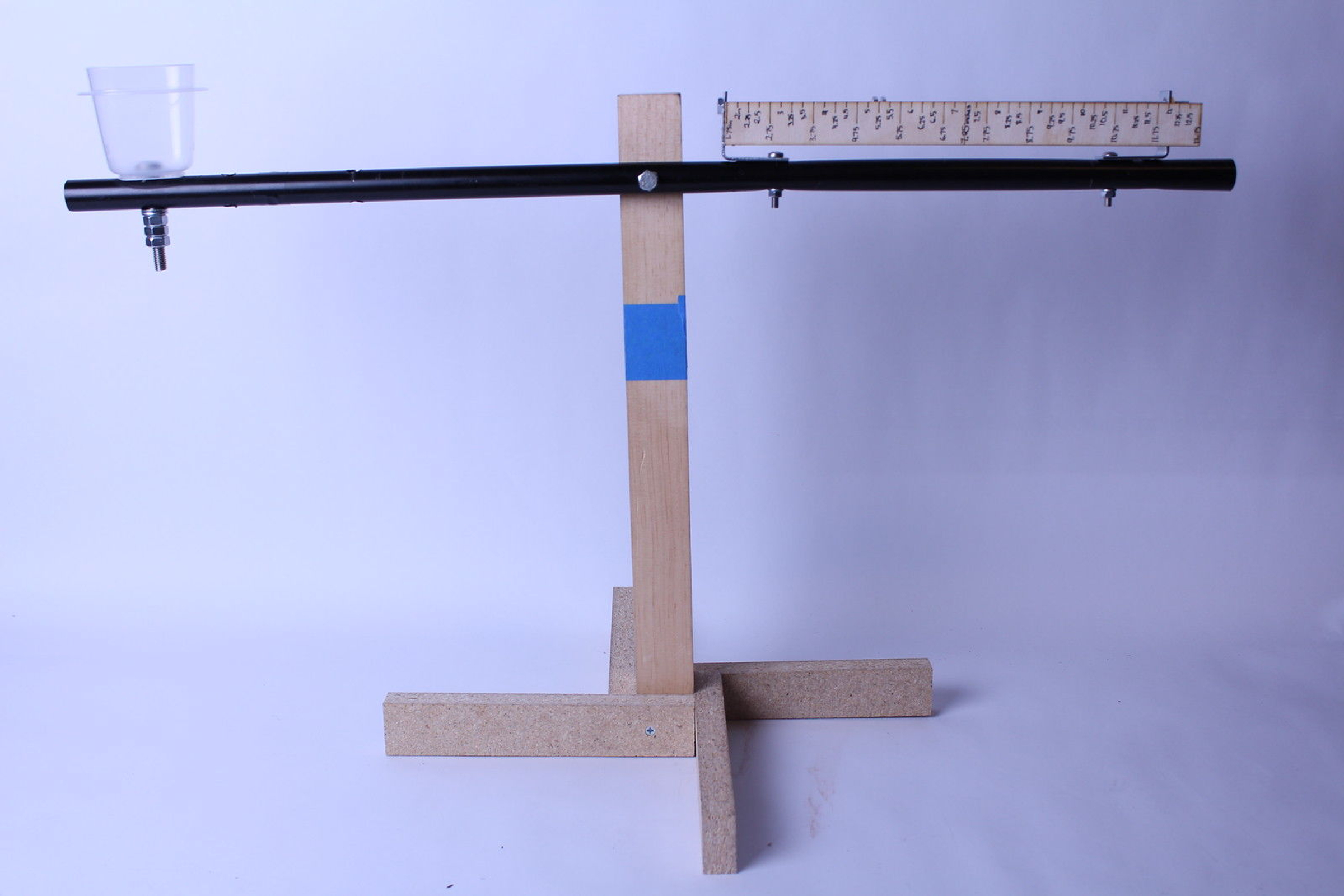# Precision Balance## Final

Shivani Angappan1 / 6

This precision balance is made entirely of commonly-found material, and was designed and built without the use of any CAD software. It is a torque balance, operated as follows:

First, one places the mass one wishes to measure in the plastic holder on the left side of the balance. Then, the nuts on the threaded rod on the right side of the balance are moved along the rod, to the right if the non-calibrated mass is too heavy, and to the left if the non-calibrated mass is too light. Then, the radial distance of the nuts is found by averaging all the distances of the nuts from the fulcrum (this can be read straight off the attached ruler). Then, one performs the math below in order to obtain the mass of the non-calibrated object.

Here's the math necessary to work the balance.

First off, let's call the mass on the left side of the plank, the mass that we want to measure, m_n, for non-calibrated mass. Then, the force of gravity on this mass is F_n = m_n * g, so the torque of this object relative to the fulcrum of the balance is T_n = F_n * r_n, where r_n is the radial distance relative to the fulcrum. We know that r_n is a fixed constant, because we want the non-calibrated mass to be right in the center of the left side of the plank. We can say that the plank has length R, so r_n  = 0.5R. Then, we know that T_n = F_n * 0.5R = m_n * g * 0.5R.

Now, let's look at the side with the calibrated mass. The way I designed the balance is for it to have movable nuts on a threaded rod, so the total torque on the calibrated mass side is the average of the torque of each separate nut, so if we let the mass of each nut be m_i and the radial distance of each nut be r_i (where 0 < i <= total number of nuts), then the total torque on the calibrated mass side is g * (∑_[i = 1]^[number of nuts] {m_i * r_i})/(number of nuts) = T_c.

In order for the calibrated mass and the non calibrated mass to be perfectly equal, we need to have the torques be equal. Thus, we need to equate our expression for T_c and T_n.

Now, we have that T_c = T_n, so g * (∑_[i = 1]^[number of nuts] {m_i * r_i})/(number of nuts) = m_n * g * 0.5R.

All of the quantities in that newest equation are defined except for m_n, so we solve for that.

Thus, we have that m_n = (g * (∑_[i = 1]^[number of nuts] {m_i * r_i})/(number of nuts)) / (0.5 R).

So, to operate the balance just right so that the masses are balanced, we need to adjust the cart on its slider along the track so that its radial distance relative to the fulcrum makes the equation come out just right. Thus, all someone would need to do to operate the balance is move the slider until the two sides of the plank are perfectly balanced (to the naked eye, which means that they will only be *very close* to perfectly balanced), and then solve the equation for m_n.## Process

Shivani Angappan1 / 17

Kepler University, located in Rwanda, is a relatively new private university that has partnered with MIT in order to further its students' education. Unfortunately, in Rwanda, science equipment is rather hard to come by, and when it is available, it is very expensive due to fluctuating inflation rates. Thus, MIT took on the challenge to create DIY science equipment for the students of Kepler. One of the most common-- and the most important--laboratory instruments is a precision balance. I chose to create one from easy-to-find materials for the benefit of the Kepler students.

### Iteration 1:

The first iteration of my balance is a simple balance with some upgrades. Like a normal balance scale, it consists of a plank with a fulcrum in the center. However, the left side of the plank has a track in it for a cart to move left and right. This is to even out the torques of the two masses on either side. This iteration was modeled on a picture of a balance that does not have the problem of a plank balancing on a 2-dimensional line, which would be the case if the fulcrum were a perfect right triangular prism. The model for this iteration had a toilet paper roll stand, with a pencil for the fulcrum (the friction produced by the pencil was very large and very inconvenient), and a toilet paper roll for the balance plank. A track was cut into the roll and a piece of cardboard, glued to another piece perpendicular to the first one, was place into the track. The friction on the track was also an obvious problem.

### Iteration 2:

This iteration of my balance was a slight renovation of the first iteration. A toilet paper roll was used for the stand again, but this time it was reinforced with even more cardboard. Instead of using a pencil for the fulcrum, I used a screw that started out smooth and cylindrical but then tapered to become serrated. This reduced the amount of friction between the balance “rod” and the fulcrum. I also used a CD cover for the base, which allowed for a small amount of stability, but not as much as desired. For the weight-system side of the balance, I used two L-shaped supports as the basis of the system. A threaded rod went through the top hole of each L-shaped support, and was held in place with a nut on either side. Several nuts were placed onto the threaded rods (to be used as calibrated weights) before fixing the rod in place with end-nuts.